### Example 83.14 Computing Sample Size for Survival Data

This example illustrates sample size computation for survival data when the accrual is not uniform and when the data contain individual loss to follow up.

Suppose that a clinic is conducting a study of the effect of a new cancer treatment. The study consists of exposing mice to a carcinogen and randomly assigning them to either the control group or the treatment group. The event of interest is death from cancer induced by the carcinogen, and the response is the time from randomization to death.

Following the derivations in the section Test for Two Survival Distributions with a Log-Rank Test, this example uses the hypothesis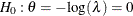with an alternative hypothesis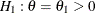, whereis the hazard ratio between the treatment group and the control group.

Suppose that from past experience, the median survival time for the control group is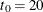weeks, and the study wants to detect a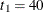weeks’ median survival time with a 80% power in the trial. If exponential survival functions are assumed for the two groups, the hazard rates can be computed from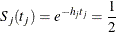where j = 0, 1.

Thus, with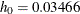and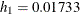, the hazard ratio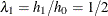, and the alternative reference is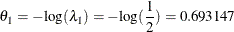The following statements invoke the SEQDESIGN procedure:

proc seqdesign altref=0.693147
;
OBrienFleming: design nstages=4
method=obf
;
samplesize model=twosamplesurvival
( nullhazard=0.03466
accrual=exp(parm=-0.1)
loss=exp(hazard=0.05)
acctime=20);
run;


The ALTREF= option specifies the alternative reference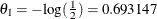. The DESIGN statement requests a group sequential design, and the METHOD=OBF option specifies the O’Brien-Fleming design. By default, the design has a two-sided alternative hypothesis in which early stopping in the interim stages occurs to reject the null hypothesis. The SAMPLESIZE statement derives required sample sizes for the test, and the MODEL=TWOSAMPLESURVIVAL option specifies a log-rank test to compare two survival distributions for the treatment effect.

The Design Information table in Output 83.14.1 displays design specifications and four derived statistics: the actual maximum information, the maximum information, the average sample number under the null hypothesis (Null Ref ASN), and the average sample number under the alternative hypothesis (Alt Ref ASN).

The SEQDESIGN Procedure
Design: OBrienFleming

Statistic Distribution Normal
Boundary Scale Standardized Z
Alternative Hypothesis Two-Sided
Early Stop Reject Null
Method O'Brien-Fleming
Boundary Key Both
Alternative Reference 0.693147
Number of Stages 4
Alpha 0.05
Beta 0.1
Power 0.9
Max Information (Percent of Fixed Sample) 102.2163
Max Information 22.35452
Null Ref ASN (Percent of Fixed Sample) 101.5728
Alt Ref ASN (Percent of Fixed Sample) 76.7397

The Boundary Information table in Output 83.14.2 displays the information level, including the proportion, actual level, and corresponding number of events at each stage. The table also displays the lower and upper alternative references, and the lower and upper boundary values at each stage.

Output 83.14.2: Boundary Information

Boundary Information (Standardized Z Scale)
Null Reference = 0
_Stage_   Alternative Boundary Values
Information Level Reference Lower Upper
Proportion Actual Events Lower Upper Alpha Alpha
1 0.2500 5.58863 22.35452 -1.63862 1.63862 -4.04859 4.04859
2 0.5000 11.17726 44.70904 -2.31736 2.31736 -2.86278 2.86278
3 0.7500 16.76589 67.06356 -2.83817 2.83817 -2.33745 2.33745
4 1.0000 22.35452 89.41808 -3.27724 3.27724 -2.02429 2.02429

In the SAMPLESIZE statement, the MODEL=TWOSAMPLESURVIVAL option specifies a log-rank test to compare two survival distributions for the treatment effect. The NULLHAZARD=0.03466 option specifies null hazard rates for the two groups under the null hypothesis. The ACCTIME= option specifies the accrual time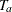, and the ACCRUAL=EXP(POWER=–0.1) option specifies that the individual accrual is truncated exponential with a scaled power parameter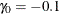. That is, the truncated exponential accrual has a power parameter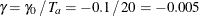in the accrual time. The LOSS=EXP(HAZARD=0.05) option specifies an exponential loss function with a hazard rate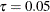. Since the ACCNOBS= option is not included with the specified accrual time, the minimum and maximum sample sizes are derived from the specified accrual time. See the section Test for Two Survival Distributions with a Log-Rank Test for a detailed derivation of these required sample sizes.

The Sample Size Summary table in Output 83.14.3 displays parameters for the sample size computation. Since the ACCNOBS= option is not included with the specified accrual time, the minimum and maximum sample sizes are derived.

Output 83.14.3: Sample Size Summary

Sample Size Summary
Test Two-Sample Survival
Null Hazard Rate 0.03466
Hazard Rate (Group A) 0.01733
Hazard Rate (Group B) 0.03466
Hazard Ratio 0.5
log(Hazard Ratio) -0.69315
Reference Hazards Alt Ref
Accrual Truncated Exponential with Losses
Scaled Exponential Parameter -0.1
Loss Hazard Rate 0.05
Accrual Time 20
Min Accrual Sample Size 269
Max Accrual Sample Size 553
Max Number of Events 89.41808

The Numbers of Events (D) table in Output 83.14.4 displays the required number of events and corresponding information level at each stage.

Output 83.14.4: Derived Sample Sizes

Numbers of Events (D)
Two-Sample Log-Rank Test
_Stage_ D Information
1 22.35 5.5886
2 44.71 11.1773
3 67.06 16.7659
4 89.42 22.3545

With the minimum and maximum sample sizes of 269 and 553, respectively, the ACCNOBS=360 option specifies an accrual sample size of 360 for the trial.

proc seqdesign altref=0.693147
;
OBrienFleming: design nstages=4
method=obf
;
samplesize model=twosamplesurvival
( nullhazard=0.03466
accrual=exp(parm=-0.1)
loss=exp(hazard=0.05)
accnobs=360 acctime=20);
run;


With the specified accrual sample size and accrual time, the Sample Size Summary table in Output 83.14.5 also displays the follow-up time and maximum sample size with the specified accrual time.

Output 83.14.5: Sample Size Summary

The SEQDESIGN Procedure
Design: OBrienFleming

Sample Size Summary
Test Two-Sample Survival
Null Hazard Rate 0.03466
Hazard Rate (Group A) 0.01733
Hazard Rate (Group B) 0.03466
Hazard Ratio 0.5
log(Hazard Ratio) -0.69315
Reference Hazards Alt Ref
Accrual Truncated Exponential with Losses
Scaled Exponential Parameter -0.1
Loss Hazard Rate 0.05
Accrual Time 20
Follow-up Time 9.100324
Total Time 29.10032
Max Number of Events 89.41808
Max Sample Size 360
Expected Sample Size (Null Ref) 359.7605
Expected Sample Size (Alt Ref) 342.9205

The Number of Events (D) and Sample Sizes (N) table in Output 83.14.6 displays the required time at each stage, in both fractional and integer numbers. The derived times under the heading Fractional Time are not integers. These times are rounded up to integers under the heading Ceiling Time. The table also displays the numbers of events and sample sizes at each stage.

Output 83.14.6: Number of Events and Sample Sizes

Numbers of Events (D) and Sample Sizes (N)
Two-Sample Log-Rank Test
_Stage_ Fractional Time Ceiling Time
D D(Grp 1) D(Grp 2) Time N N(Grp 1) N(Grp 2) Information D D(Grp 1) D(Grp 2) Time N N(Grp 1) N(Grp 2) Information
1 22.35 7.74 14.62 11.4005 200.79 100.39 100.39 5.5886 24.46 8.48 15.98 12 211.67 105.83 105.83 6.1162
2 44.71 15.69 29.01 17.0454 304.52 152.26 152.26 11.1773 48.97 17.23 31.75 18 322.36 161.18 161.18 12.2436
3 67.06 23.81 43.25 21.9812 360.00 180.00 180.00 16.7659 67.14 23.84 43.30 22 360.00 180.00 180.00 16.7851
4 89.42 32.39 57.03 29.1003 360.00 180.00 180.00 22.3545 91.46 33.21 58.25 30 360.00 180.00 180.00 22.8649